# JavaScript 进阶之高阶函数篇### 高阶函数定义

1. 函数可以作为参数传递到另外一个函数执行
2. 函数可以作为返回值输出被执行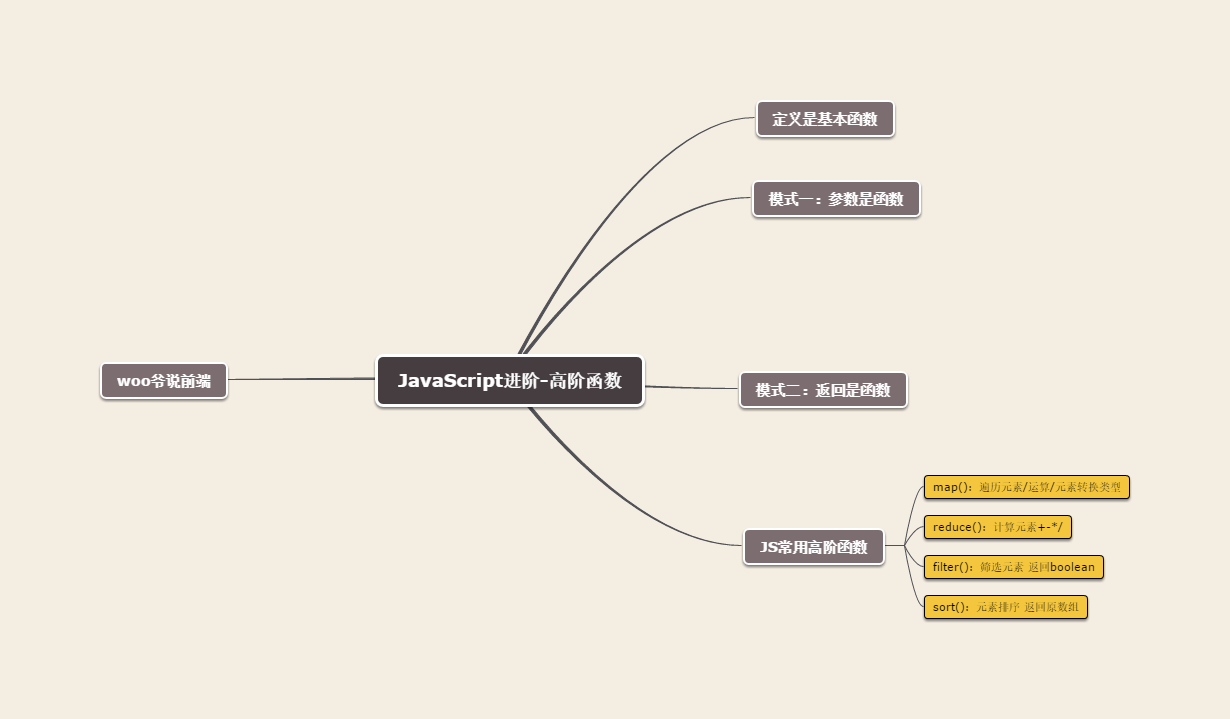### 第一种模式：作为参数传递

// 两个不同的函数，但是其中一部分逻辑是相同的一部分是可变的     function a(){    console.log(" 我是一个函数 ")；    console.log(" 我是 a 函数 ")；          }     function b(){   console.log(" 我是一个函数 ");   console.log(" 我是 b 函数 ")     }  /* 以上就是我们两个基本得函数，我们分别执行这两个函数 */  a()； b()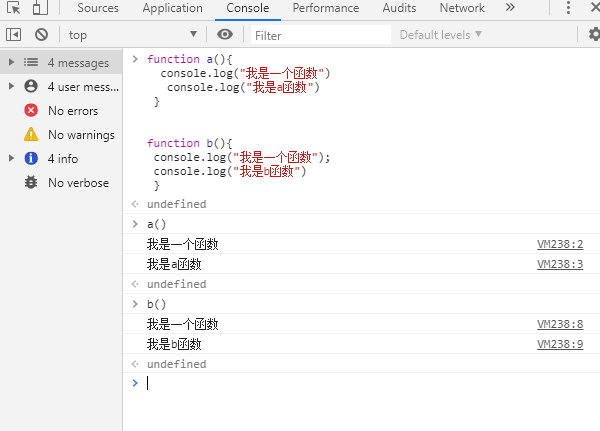function c(fn){ console.log(" 我是一个函数 ")  fn()  } // 把相同的逻辑封装成一个 c 函数，不同逻辑的作为 fn 参数函数传递进去执行 c(function(){   console.log(" 我是 a 函数 ") }) c(function(){   console.log(" 我是 b 函数 ") }) // 由此可见我们实现我们想要的呈现方式，接下来我们看一下执行的结果是怎么样的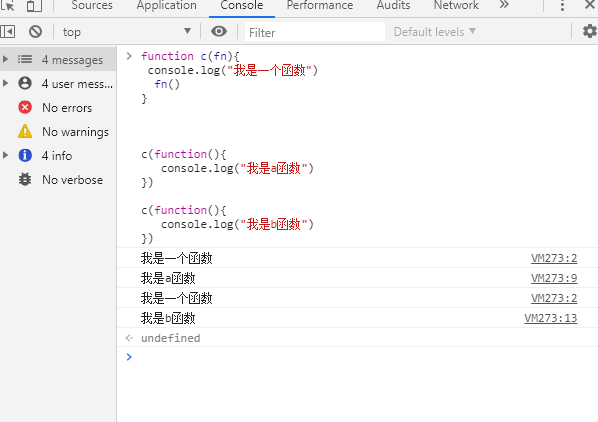var httpsAjax = function(obj,callback){    var {url,data,type} = obj;    \$.ajax({        url:url,        type:type || 'POST' ,        data:dara || {},        success:function(res){          // 利用 typeof 判断数据类型，如果是传进来的是函数, 我们就执行回调函数          if(typeof callback === 'function'){             callback(res)          }        }      })}     httpsAjax({        url:"xxx/get/user",        type:"GET",        data:{}    },function(res){      // 操作一定的业务逻辑      console.log(res)   })

### 第二种模式：作为返回值输出

var isString = function(obj){  return object.prototype.toString.call(obj) === '[object String]'  }  var isArray = function(obj){  return object.prototype.toString.call(obj) === '[object Array]' } var isNumber = function(obj){  rturn object.prototype.toString.call(obj) === '[object Number]'  }  isString(" 我是一个数组串 ")；//trueisArray(["1","2"]);//trueisNumber(1)//true

var is_type = function(obj,type){  return object.prototype.toString.call(obj) == '[object ' + type + ']'  } console.log(is_type(11,'Number'));//trueconsole.log(is_type(['a','b'],"Array");//true

var isType = function(type){    // 先传递一个 type 参数作为数据类型，利用高阶函数拥有保存变量的特性，返回一个可持续执行的函数    return function(obj){          return object.prototype.toStirng.call(obj) === '[object '+ type +']'          }} // 先细分到每种类型，这样我们就可以明确知道具体类型调用什么方法 var isString = isType("String"); var isArray = isType("Array"); var isNumber = isType("Number"); console.log(isArray([1,2,3]))//true

### map() 方法

1. 定义：map() 方法遍历原有数组返回一个新数组，数组中的元素为原始数组元素调用函数处理后的值，按照原始数组元素顺序依次处理元素。
2. 注意：不会对空数组进行检测、返回的是新数组不会改变原始的数组
3. 语法格式 ：

newArray.map(function(item){     // 遍历 newArray item 是 newArray 元素；类似于 newArray[i]　　return item　　// 必须有 return，反正不会产生新的数组 })

map() 方法定义在 JavaScript 的 Array 中，我们调用 Array 的 map 方法，传入我们自己想要的函数，就等到一个新的 array 作为结果，例子如下：

function pow(x){  return x*x  } var arr = [1,2,3,4,5];console.log(arr.map(pow));//[1,4,9,16,25]

var arr = [    {        name:" 张三 "，        type:2    },    {         name:" 李四 ",         type:3    } ]    // 要求拿到 type 属性组成新的数组  // 以往的写法 for 循环var new_arr = []for(var i  = 0 ; i < arr.length ; i++){    var obj = arr[i]    for(var key in obj){       if(key == 'type'){           new_arr.push(obj[key])        }    } } //map 的写法 var new_arr = arr.map(function(item){     return item.type    }) 

### reduce() 方法

1. 定义：接收一个函数作为累加器，数组中的每一个值（从左到右）开始遍历，最终计算为一个值
2. 注意：对空数组是不会执行回调函数的
3. 语法格式 : new Array.reduce(callback,initialValue)

• reduce(callback,initialValue) 会传入两个变量，第一个参数是回调函数（callback）和第二个初始值（initialValue）。
• 第一个参数回调函数（callback）有四个传入参数，prev 和 next，index 和 array。prev 和 next 是必传的参数。
• 第一个参数初始值（initialValue）决定回调函数的第一个参数 prev 的取值结果，当 reduce 传入 initialValue 时，prev 的默认值就是 initialValue，当 reduce 没有传入 initialValue 时，那么 prev 的默认值就是原数值的第一个元素值。

var arr = ["apple","orange"]; // 第一种没有传递 initialValue 的情况function getValue(){  return arr.reduce(function(prev,next){        console.log("prev",prev);        console.log("next",next)        return prev;    })}  console.log("getValue",getValue())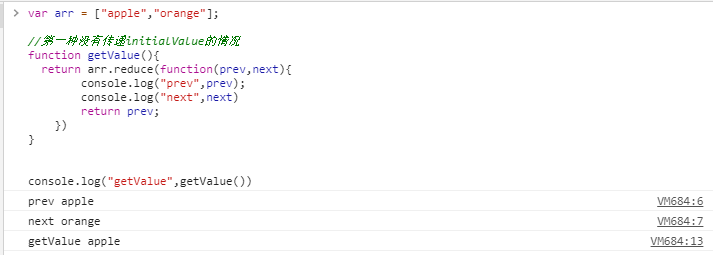var arr = ["a","b"];  // 传递 initialValue 情况时 function getValue(){    return arr.reduce(function(prev,next){       console.log("prev",prev);       console.log("next",next);       prev[next] =1;       return prev;       },{})    //initialValue 传递一个空对象} console.log("getValue",getValue());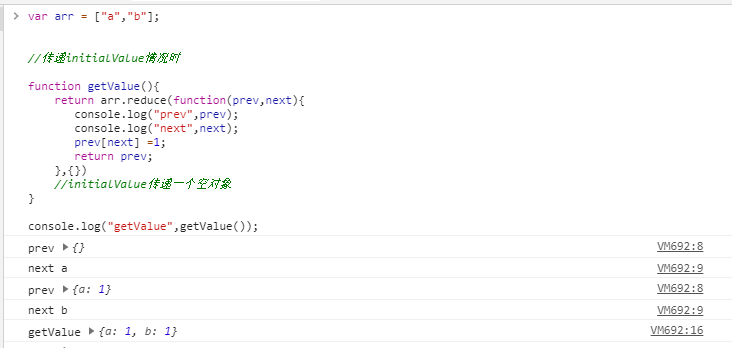var numArray = [1,2,3,4,5];  // 用 for 循环来计算var num = 0; for(var i = 0 ; i < numArray.length ; i++){   num = num + numArray[i]  } console.log(num);//15 // 利用 reduce 方法 var res = numArray.reduce(function(prev,next){    return prev + next},0) console.log(res) ;//15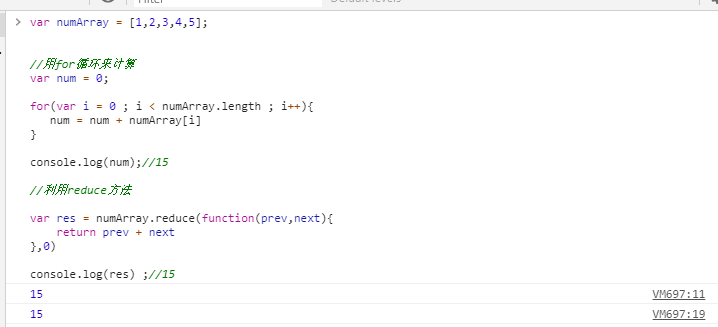var arr = [[0,1],[2,3],[4,5]]; var res = arr.reduce(function(prev,next){    return prev.concat(next)},[]) console.log(res);//[0,1,2,3,4,5];

var arr = ["apple","orange","apple","orange","pear","orange"];  // 不用 reduce 时 function getWorad(){  var obj = {};  for(var i = 0 ; i < arr.length ; i++){    var item = arr[i];    if(obj[item]）{      obj[item] = obj[item] + 1    }else{      obj[item] = 1    }  }  return obj} console.log(getWorad()); function getWorad2(){  return arr.reduce(function(prev,next){    prev[next] = (prev[next] + 1 ) || 1;    return prev;  },{})}console.log(getWorad2())

### filter() 方法

1. 定义：filter() 也是一个常用的操作，它用于把 Array 的某些元素过滤调，然后返回剩下的元素，和 map 方法类似，Array 的 filter 也接收一个函数，和 map() 不同的是；filter() 把传入的函数依次作用于每个元素，然后根据返回值是 true 还是 false 决定保留还是丢失该元素

var arr = [1,2,3,4,5]; var r = arr.filter(function(item){   return item%2 !== 0 ;}) console.log(r)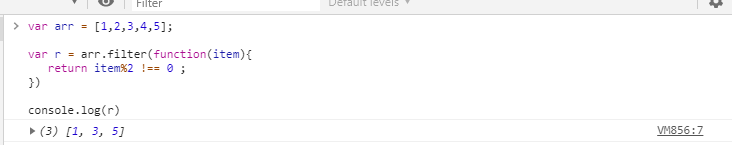var arr = ['a','b','','c']var r = arr.filter(function(item){   return item && item.trim();}) console.log(r)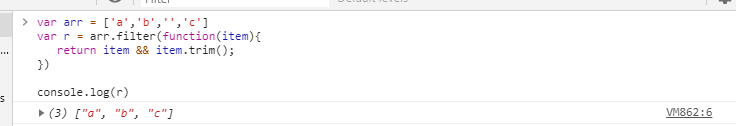// 先看一下 filter 的语法格式var r = Array.filter(function(ele,index,_this){    console.log(ele);    console.log(index);    console.log(_this);    return ture;}) /*{1}可见用 filter() 这个高阶函数，关键在于正确实现一个筛选函数回调函数：filter() 接收的回调函数，其实可以有多个参数。通常我们仅使用第一个参数，表示 Array 的某个元素，回调函数还可以接收另外两个参数，表示元素的位置，和数组本身。{1}*/ 　　业务中最经常用到的还是用 filter 来去除数组中重复的元素var r,arr = [1,1,3,4,5,3,4]; r= arr.filter(function(ele,index,self){   return self.indexOf(ele) === index;}) console.log(r)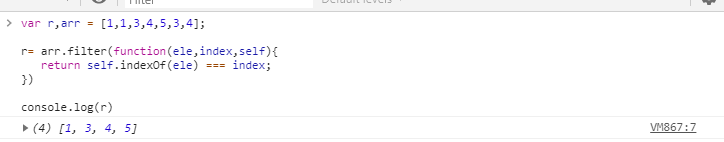### sort() 方法

1. 定义：sort() 方法用于对数组的元素进行排序，并返回数组。
2. 语法格式：arrayObject.sort(sortby);
3. 注意：参数 sortby 可选，用来规定排序的顺序，但必须是函数。

// 接下来我们就来看一下到底如何排序 // 从小到大的排序，输出：[1,2,3,9,56,87]var arr = [9,87,56,1,3,2];arr.sort(function(x,y){   if(x < y){      return -1   }   if(x > y){      return 1   }      return 0;}) // 如果我们想要倒序排序；我们可以把大的放在前面 [5,4,3,2,1]var arr = [1,2,3,4,5];arr.sort(function(x , y){     if(x < y ){        return 1     }     if(x > y){        return -1     }      return 0}) // 在比如我们想要对字符串排序也可以实现，以字符串的首个字符，按照 ASCII 的大小    // 进行比较的，忽略大小写字母 ['good','apple','moide'] var arr = ['good','apple','moide']; arr.sort(function(s1,s2){    var x1 = s1.toUpperCase();// 转成大写    var x2 = s2.toUpperCase();    if(x1 < x2){       return -1     }     if(x1 > x2){        return 1     }     return 0})// 忽略大小写其实是把值转成大写或者全部小写，再去做比较 // 注意：sort() 方法会直接对原有的 Array 数组进行修改，他返回的结果仍是当前的 Array    // 还有往往我们会在代码里见到很多数组对象，相对数组对象的某个属性做排序那要怎么实现呢// 下面就是对数组对象的排序例子 var arr = [  {     Name:'zopp',     Age:10   },   {      Name:'god',      Age:1,    },    {       Name:'ytt',       Age:18     }]; // 简单的写法 ; 默认的升序 function compare(prototype){ return function(a,b){        var value1 = a[prototype];        Var value2 = b[prototype];        return value1 - value2}}// 一般我们往往会把参数函数单独写一个函数；达到清晰明确，多变性 console.log(arr.sort(compare(“Age”)));/* 最后我们写了这么多例子，也发现作为可变的部分参数函数其实无非就是两种；一种是升序一种是降序。其实我们还可以把这可变的参数函数封装成一个方法，利用传参的方式来说明我们是要升序还是降序*//** 数组根据数组对象中的某个属性值进行排序的方法      * 使用例子：newArray.sort(sortBy(type,rev,[,key]))      * @param type 代表 newArray 的类型，’number’表示数值数组 [1,2]，’string’表示字符串数组 [‘abhs’,’as’]，’obj’表示对象数组 [{},{}]，如果是 obj 的话第三个参数必填     * @param rev true 表示升序排列，false 降序排序     * @param key 非必填的第三个函数，是作为如果 type 是 obj 的话作为属性传递     * */ var sortBy = function(type,rev,key){    // 第二个参数不传默认就是升序排列    if(!rev){     rev = 1   }else{     rev = rev ? 1 : -1;    }return function(a,b){  // 如果是 string 的话我们就要处理一下统一大写还是小写   if(type == 'string'){     a = a.toUpperCase();     b = b.toUpperCase();  }  // 如果是 obj 的话我们就是取对应的属性值  if(type == 'obj'){     a = a[key];     b = b[key];  }   if(a < b){     return rev * -1;   }   if(a > b){     return rev * 1;   }    return 0;    }} // 这就是我们最后想要的统一封装，大家也可以拿着自己去修改一下，这种封装是最后返回一个处理好的匿名函数，也是高阶函数中的一种，后面我们也会提到。

• 比较函数应该具有两个参数 a 和 b，其返回值如下：
• 若 a 小于 b，即 a - b 小于零，则返回一个小于零的值，数组将按照升序排列。
• 若 a 等于 b，则返回 0。
• 若 a 大于 b, 即 a - b 大于零，则返回一个大于零的值，数组将按照降序排列。